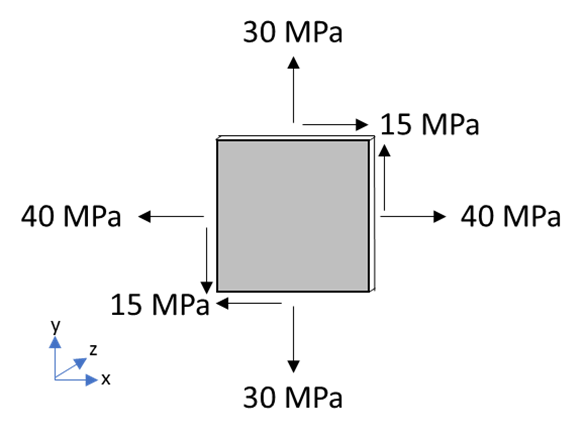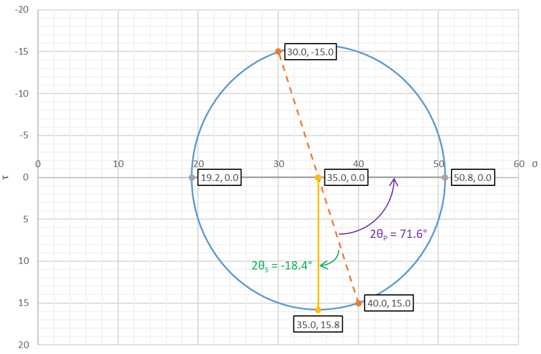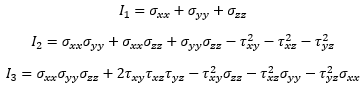top of page
Search

# Mohr’s Circle – What Is It And Why Should We Know It?

Most mechanical engineers in their studies will learn Mohr’s Circle. It’s an important concept that, unfortunately, isn’t appreciated much outside the university curriculum. But what is it and can we apply it to our understanding of FEA results? Read further to find out!

## A Quick Background

Mohr’s circle is named after its creator, Christian Otto Mohr, who developed the method in the late 1800s. It built off the previous concepts of the Cauchy stress tensor and Karl Kulmann’s method of graphically visualizing stresses.

Mohr’s circle is a way to visualize the transformation equations for plane stress. For a more detailed explanation on what plane stress is, refer to this awesome blog post. Essentially, plane stress assumes that the three stress tensor components related to the z-direction are 0. This assumption is mainly used for plate surfaces and very thin parts.

Any engineer performing FEA should know Mohr’s circle because it is essential to understanding how normal, shear, and principal stresses interact and determining the plane in which they act. The principles used in generating Mohr’s circle are the same principles FEA softwares use. Knowing how the software works is essential; otherwise, you’re just blindly trusting the outputs – and that’s never a good idea!

## Making The Circle

Because of the plane stress assumption, there are three non-zero values within the stress tensor involved in making Mohr’s Circle: σx, σy, and τxy. These equations would give two points in a coordinate system where x is σ and y is τ: (σx, τxy) and (σy, -τxy). With two points forming the diameter, only one unique circle can be made to connect them. The idea behind Mohr’s circle is that any point on the circle is a valid stress state of the element depending on its orientation, θ. Below are the equations used to determinate the center point and radius of the circle.The center point is represented by the point (σavg,0). Then, the radius value can be used around the center point to draw Mohr’s circle.

We will illustrate this using an example. Say we have a stress element where σx = 40 MPa, σy = 30 MPa, and τxy = 15 MPa. By using the above equations, we find that σavg = 35 MPa and R = 15.8 MPa. We will draw the Mohr’s circle below:## How To Use It

In Mohr’s circle, the x-axis is σ and the y-axis is τ. In order to keep the standard angle convention of counterclockwise being positive, the y-axis is reversed.

The minimum and maximum principal stresses as well as the maximum shear stress can be determined from initial inspection of Mohr’s circle. As shown in gray, the maximum and minimum principal stresses are determined using σavg ± R. The maximum shear stress, shown in yellow, is determined by R. The initial stress state is shown in orange.

These points are loaded at a certain orientation that can be determined by the following equations:We can calculate these orientations using our example to be θP = 35.8° and θS = -9.2°. This means if you rotate the original stress element 35.8 degrees, then you will achieve the maximum principal stress. If you rotate the original stress element -9.2 degrees, then you will achieve the maximum shear stress.

Additionally, you can determine the principal and shear stresses at any angle by the following equations:Note that the angle is measured from the initial stress state and NOT from the x or y axis. So, if you had 0 as the angle, σx1 would be 40 MPa and τx1y1 would be 15 MPa, which is the untransformed stress state.## Does It Work In 3 Dimensions?

Yes it does – you just have to make more circles (more circles = Mohr circles, lololol)! Essentially, you would have six possible non-zero values in the stress tensor and would make three Mohr’s circles – one in the xy plane, one in the xz plane, and one in the yz plane. In order to do this, the principal stresses must first be calculated by solving for the roots of the below polynomial:whereOnce the three roots are found (which we will call σ1, σ2, and σ3), then the centers and radii of the three Mohr’s circles can be found using the below equations where σ1 ≥ σ2 ≥ σ3:The final result should be two circles inscribed within a third circle, corresponding to the plane stress states of the three principal stresses, and you can do stress transformations along the principal axes for any of the three circles.

Any stress within the shaded region in the 3D Mohr’s circle is a valid combination of normal and shear stresses in the stress element.There are ways to determine the normal and shear stresses on a certain plane within the 3D stress element as well as deriving the plane from a certain combination of normal and shear stresses, but they don’t need to involve the Mohr’s circle, so we will stop here for now and end with an example of drawing the 3D Mohr’s circle.

For our example, let’s use the stress tensorWe can calculate the polynomial and the principal stresses to beNow we can calculate C and R for each circle and draw the 3D Mohr’s circle:All values outside of the yellow and blue circles but within the orange circle are valid combinations of normal and shear stresses.

## Final Thoughts

Mohr’s circle is a great tool for visualizing stress transformations in plane stress elements. It can also be applied to strains and moments using the same methodology.

Our engineering team loves spending their free time drawing Mohr’s Circle and we’d be happy to help you with any mechanics related project, whether that be FEA or CFD. Reach out to us today!

3,606 views
bottom of page